Students can access the CBSE Sample Papers for Class 11 Maths with Solutions and marking scheme Term 2 Set 2 will help students in understanding the difficulty level of the exam.

## CBSE Sample Papers for Class 11 Maths Term 2 Set 2 with Solutions

Time: 2 Hours
Maximum Marks: 40

General Instructions:

• This question paper contains three sections A, B and C. Each part is compulsory.
• Section -A has 6 short answer type (SA1) questions of 2 marks each.
• Section -B has 4 short answer type (SA2) questions of 3 marks each.
• Section -C has 4 long answer type questions (LA) of 4 marls each.
• There is an internal choice in some of the questions.
• Q14 is a case-based problem having 2 sub parts of 2 marks each.

Section – A

Question 1.
A wheel makes 360 revolutions in 1 min. Through how many radians does it turn in 1 second.
OR
Find the degree measure of the angle subtended at the centre of circle of radius 100 cm by an arc of 22 length 22 cm. (Use π = $$\frac{22}{7}$$)
No. of revolution in one minute = 360
∴ No. of revolutions m one second = $$\frac{360}{2}$$
= 6

∴ 6 revolutions = 6 × 2π = 12π radians
Hence, no. of radians turned in one second = 12π
OR
Given, l = length of arc = 22 cm
r = radius of circle = 100 cm
Let θ be the angle subtended at the centre, then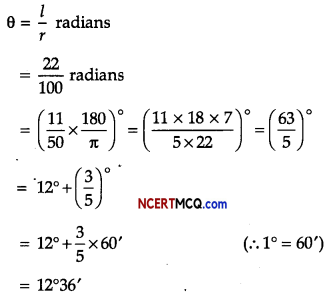Question 2.
If parabola y2 = px passes through point (2, -3), find the length of latus rectum. (2)
Given equation of parabola is y2 = px …(i)
Since (i) passes through (2, -3).
∴ (-3)2 = p.2
⇒ p = $$\frac{9}{2}$$
∴ y2 = $$\frac{9}{2}$$x
⇒ y2 = 4. $$\frac{9}{8}$$x
Comparing above by y2 = 4ax
a = $$\frac{9}{8}$$
∴Length of latus rectum = 4a = $$\frac{9}{2}$$ units

Students make mistakes while comparing the given equation with the general form of parabola.

Learn the equations of parabola and the terms released it. Understand the equation and its properties with the help of its diagram.Question 3.
How many 5 digit telephone numbers can be constructed using the digits 0 to 9, if each number starts with 67 and no digit appears more than once?
OR
Determine the number of 5 card combining out of deck of 52 cards if each selection of 5 cards has exactly one king. (2)
Number of ways to fill the III place = 8
Number of ways to fill the IV place = 7Number of ways to fill the V place = 6
Hence, total number of ways = 8 × 7 × 6 = 56 × 6 = 336
OR
1 king out of 4 kings can be selected in 4C1 ways
Remaining 4 cards out of remaining 48 cards can be selected in 4C4 ways.
Hence, total number of ways
= 4C1 × 48C4 = 4 × 48C4

Question 4.
Given P(A) = $$\frac{3}{5}$$ and P(B) = $$\frac{1}{5}$$. Find P(A ∪ B) if A and B are mutually exclusive events. (2)
P(A) = $$\frac{3}{5}$$, P(B) = $$\frac{1}{5}$$
A and B are mutually exclusive events
∴ P(A ∩ B) = 0
Now P(A ∪ B) = P(A) + P(B) – P(A ∩ B)
= $$\frac{3}{5}+\frac{1}{5}$$ – 0
P(A ∪ B) = $$\frac{4}{5}$$ = 0.8

Question 5.
Solve the inequality: -3 ≤ 4 – $$\frac{7x}{2}$$ ≤ 18 (2)
The given inequality -3 ≤ 4 – $$\frac{7x}{2}$$ ≤ 18 Adding (-4) to each term,
-3 – 4 ≤ 4 – $$\frac{7x}{2}$$ – 4 ≤ 18 – 4
-7 ≤ – $$\frac{7x}{2}$$ ≤ 14

Multiplying by ($$\frac{-2}{7}$$) to each term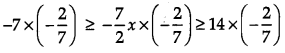⇒ 2 ≥ x ≥ -4
⇒ 4 ≤ x ≤ 2
∴ Solution set = [-4, 2]

Question 6.
Find the derivatives of 2x – $$\frac{3}{4}$$ (2)
Let f(x) = 2x – $$\frac{3}{4}$$
∴ f'(x) = $$\frac{d}{d x}$$(2x – $$\frac{3}{4}$$)
= 2$$\frac{d}{d x}$$x – $$\frac{d}{d x}$$($$\frac{3}{4}$$)
= 2 × 1 – 0
= 2

Section – B

Question 7.
Find the centre and radius of the circle 2x2 + 2y2 – x = 0. (3)
Given equation of the circle is
2x2 + 2y2 – x = 0Question 8.
Prove that: $$\frac{\cos 4 x \sin 3 x-\cos 2 x \sin x}{\sin 4 x \cdot \sin x+\cos 6 x \cdot \cos x}$$ = tan 2x (3)
OR
Prove that:
tan α. tan (60° – α) . tan (60° + a) = tan 3α (3)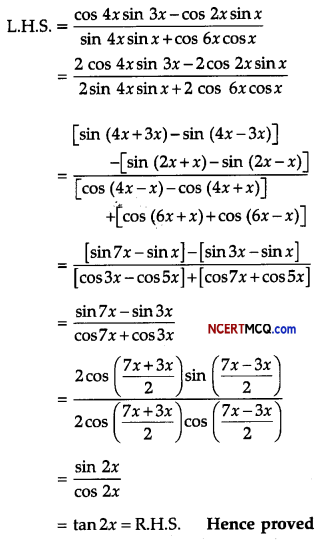Many students get confused among the formula of trigonometry. They must learn all the formulae properly and practise the derivation of the formula to avoid mistake.

Simplification of trigonometric equations needs sufficient practice. Give ample practice such type of questions.

OR

L.H.S = tan α. tan (60° – α). tan (60° + a)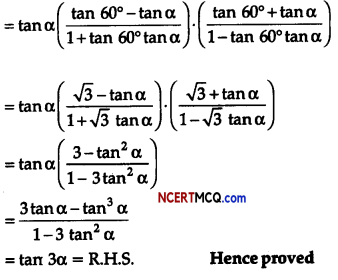Question 9.
From a class of 40 students, in how many ways can five students be chosen for an excursion party. (3)
From a dass of 40 students, five students can be chosen for an excursion party in 4C5 ways= 658,008

Question 10.
Find the value of the expression
3[sin4($$\frac{3 \pi}{2}$$ – α) + sin4(3π + α)] – 2[sin6($$\frac{\pi}{2}$$ + α) + sin6(5π – α)]
Given 3[sin4($$\frac{3 \pi}{2}$$ – α) + sin4(3π + α)] – 2[sin6($$\frac{\pi}{2}$$ + α) + sin6(5π – α)]

= 3[cos4α + sin4(π + α)] – 2[cos6 α + sin6(π – α)]
= 3(cos6α + sin6α] – 2[cos6 α + sin6α]
= 3(cos4α + sin4 α + 2sin2α cos2α – 2sin2α cos2α] – 2(cos2α + sin2α)3 – 3cos2α sin2α(cos2α + sin2α)]
= 3[(cos2α + sin2α)2 – 2sin2αcos2α] -2[1 – 3cos2αsin2α]
= 3(1 – 2sin2αcos2α – 2 + 6cos2αsin2α]
= 3 – 6sin2αcos2α – 2 + 6sin2αcos2α
= 1

Section – C

Question 11.
Using first principle find the derivative of x cos x function: (4)
Let f(x) = x cos x
∴ f(x + h) = (x + h)cos(x + h)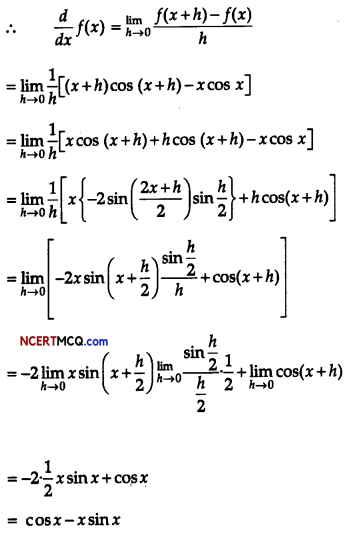Question 12.
A solution of 9% acid is to be diluted by adding 3% acid solution to it. The resulting mixture is to be more than 5% but less than 7% acid. If there is 460 L of the 9% solution, how many litres of 3% solution will have to be added?
OR
A solution is to be kept between 40°C and 45°C. What is the range of temperature in degree Fahrenheit, if the conversion formula is F = $$\frac{9}{5}$$C +32? (4)
Let x L of 3% solution be added to 460 L of 9% solution of acid.
Then, total quantity of mixture = (460 + x) L
Total add content in the (460 + x) L of mixture
= (460 × $$\frac{9}{100}$$ + x × $$\frac{3}{100}$$)L
It is given that add content in the resulting mixture must be more them 5% but less than 7%.
Therefore,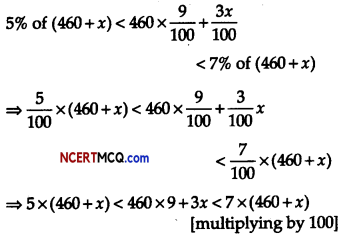⇒ 2300 + 5x < 4140 + 3x < 3220 + 7x
Taking first two inequalities,
2300 + 5x < 4140 + 3x
⇒ 5x – 3x < 4140 – 2300
⇒ 2x < 1840
⇒ x < $$\frac{1840}{2}$$
⇒ x < 920 …(i)
Taking last two inequalities,
4140 + 3x < 3220 + 7x
⇒ 3x – 7x < 3220 – 4140
⇒ -4x < -920 ⇒ 4x > 920
⇒ x > $$\frac{920}{4}$$
⇒ x > 230 …(ii)
Hence, the number of litres of the 3% solution of add must be more than 230 L and less than 920 L.

OR

Let the required temperature be x° F.
Given that, F = $$\frac{9}{5}$$C + 32
⇒ 5F = 9C + 32 × 5
⇒ 9C = 5F – 32 × 5
∴ C = $$\frac{5 F-160}{9}$$
Since, temperature in degree Celsius lies between 40°C to 45°C.
Therefore, 40 < $$\frac{5 F-160}{9}$$ < 45
40 < $$\frac{5 F-160}{9}$$ < 45
⇒ 40 × 9< 5x – 160 < 45 × 9 [multiplying throughout by 9]
⇒ 360 < 5x – 160 < 405 [adding 160 throughout]
⇒ 360 + 160 <5x< 405+160
⇒ 520 < 5x < 565 1
⇒ $$\frac{520}{5}$$ < x < $$\frac{565}{5}$$ [divide throughout by 5]
⇒ 104 < x < 113
Hence, the range of temperature in degree Fahrenheit is between 104 °F to 113°F.Question 13.
If A and B be the points (3,4,5) and (-1,3, -7), respectively, find the equation of the set of points P such that PA2 + PB2 = k2, where k is constant. (4)
The coordinates of points A and B are given as (3, 4, 5) and (-1, 3, -7) respectively. Let the co-ordinates of point P be (x, y, z).
On using distance formula, we obtain
PA2 = (x – 3)2 + (y – 4)2 + (z – 5)2
= x2 + 9 – 6x + y2 + 16 – 8y + z2 + 25 – 10z
= x2 + y2 + z2 – 6x – 8y – 10z + 50

PB2 = (x + 1)2 + (y – 3)2 + (z + 7)2
= x2 + 1 + 2x + y2 + 9 – 6y + z2 + 49 + 14z
= x2 + y2 + z2 + 2x – 6y + 14z + 59

Now, if PA2 + PB2 = k2, then
(x2 + y2 + z2 – 6x – 8y – 10z + 50) + (x2 + y2 + z2 + 2x – 6y + 14z + 59)
⇒ 2x2 + 2y2 + 2z2 – 4x – 14y + 4z + 109 = k2
⇒ 2(x2 + y2 + z2 – 2x – 7y + 2z) + 109 = k2
⇒ 2(x2 + y2 + z2 – 2x – 7y + 2z) = k2 -109
⇒ x2 + y2 + z2 – 2x – 7y + 2z = $$\frac{k^{2}-109}{2}$$
Thus, the required equation is
x2 + y2 + z2 – 2x – 7y + 2z = $$\frac{k^{2}-109}{2}$$

Case-Based/Data Based

Question 14.
A child’s game has 8 triangles of which 3 are blue and rest are red, and 10 squares of which 6 are blue and rest are red. One piece is lost at random.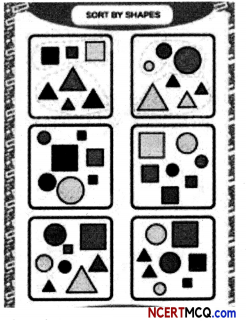(i) Find the probability that lost piece is triangle. (2)
Since, total no. of triangles = 8
Triangles with blue colour = 3
Triangles with red colour = 8 – 3 = 5 and
total no. of squares = 10
Squares with blue colour = 6
Squares with red colour = 10 – 6
= 4

Number of favourable outcomes for the event that lost figure is triangle,
i.e., F (E) = 8
Total figures (square and triangle)
= 8 + 10 = 18
i.e., T(E) = 18

Probability (getting a triangle),
P(E) = $$\frac{F(E)}{T(E)}$$
= $$\frac{8}{18}=\frac{4}{9}$$

(ii) Find the probabilities that lost piece is square of blue colour & triangle of red colour. (2)
P(E) = $$\frac{F(E)}{T(E)}$$
= $$\frac{6}{18}=\frac{1}{3}$$
P(E) = $$\frac{5}{18}$$